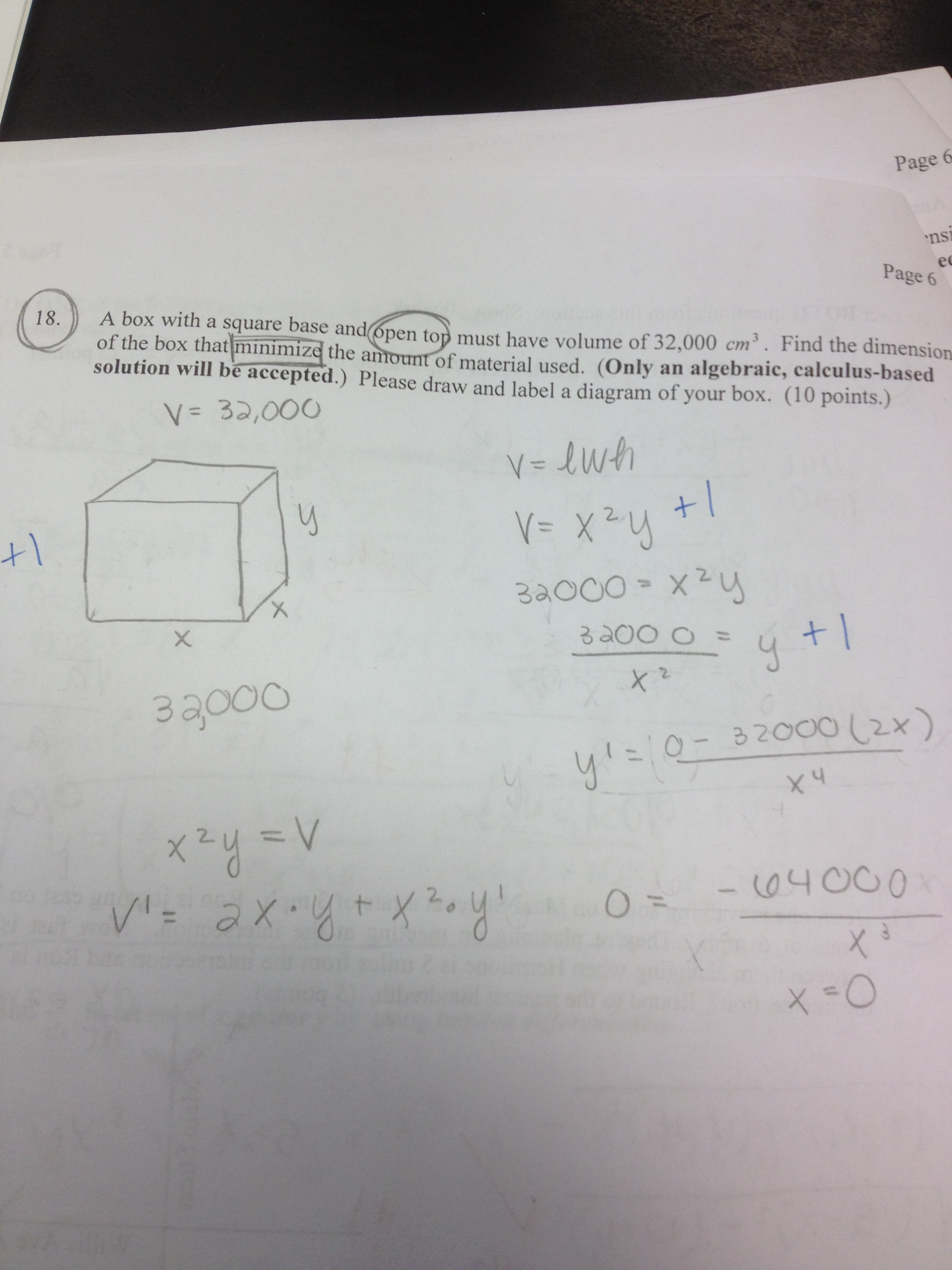Categories

# Santa’s getting cheap: Minimizing the area of a boxThis student knows stuff. What does the student know? Where did they go wrong, and why do you think they went wrong? How might you help?

## 4 replies on “Santa’s getting cheap: Minimizing the area of a box”Barry Smithsays:

The student doesn’t know the real beginning of the solution process for optimization problems:

Identify the quantity being optimized
Write a relationship involving that quantity
Possibly solve the relationship for that quantity

It is only at this point that they need the constraint that the volume is 32000. Because the constraint was written before the question in the problem statement, it looks like the student started with the constraint and then ran out of ideas.

My discussion with the student would address:
1) Reading through the whole problem before writing anything. If an optimization problem is identified, start with the process I indicated above.
2) Not writing a single word in the solution of a math problem inhibits clear thinking. On the other hand, writing words and organizing information can often aid in the process of finding a solution. I see no indication that the student understands what is meant by “the amount of material used”. Writing this phrase as part of identifying the quantity to be optimized and seeing it written while thinking about what to do next might have helped the student realize that more was needed than the volume.louisesays:

I agree. I would start by asking the student what is the material used.
I am not always so bothered by a lack of words if they are replaced by pictures: in this case I’d expect to see a picture somehow representing the 4 sides and the square base. I think if we go to that picture, we could dig ourselves out of this mess.
On the up side, this student does know how to do derivatives by parts (or whatever you call it in the USA).Jim Dohertysays:

Going out on a limb here…
I have often attacked these types of problems with a combination of algebra/calculus and the graphing calculator for support. Unfortunately, the graphing calculator only accepts equations of the form y = NOT V = or A = or any other type. Soooo, we solve for the volume by substituting for y in the 32000 = x^2 y equation then we enter it into our calculators as y = NOT as V = This change in variable name, while not troubling to those who know what’s going on, is absolutely troubling to those with fragile knowledge. I think that is what is behind the quotient rule application of y = 32000/x^2 I have seen this mistake before. The student ‘knows’ what to do here and did it pretty well. Unfortunately what the student ‘knows’ is not correct. Does this seem reasonable to any of you?

The student has lost track of the fact that he or she is trying to minimize the area, not the volume. This is a correct solution to the somewhat perverse question “how can I make a box of volume 32000 with minimal volume?” The derivative comes out to 0 because, once we have imposed the constraint, the volume is a constant function of x.

I am impressed by the skill with calculation here. This student correctly takes the derivative of V, including remembering the chain rule term y’. This student will do well when performing implicit differentiation.

My advice to this student would be, at the start of a constrained optimization problem, to write out in words: The constraint(s) is/are … I am trying to minimize/maximize …